# Scratch 平台的神经网络实现（R 语言）

Andrej Karpathy 在 CS231n 课程中这样说道 ：

“我们有意识地在设计课程的时候，于反向传播算法的编程作业中包含了对最底层的数据的计算要求。学生们需要在原始的 numpy 库中使数据在各层中正向、反向传播。一些学生因而难免在课程的留言板上抱怨（这些复杂的计算）”

import keras
model = Sequential()
model.compile(loss=’categorical_crossentropy’, optimizer=RMSprop())
model.fit()

Karpathy教授,将“智力上的好奇”或者“你可能想要晚些提升核心算法”的论点抽象出来，认为计算实际上是一种泄漏抽象（译者注：“抽象泄漏”是软件开发时，本应隐藏实现细节的抽象化不可避免地暴露出底层细节与局限性。抽象泄露是棘手的问题，因为抽象化本来目的就是向用户隐藏不必要公开的细节--维基百科）：

“人们很容易陷入这样的误区中-认为你可以简单地将任意的神经层组合在一起然后反向传播算法会‘令它们自己在你的数据上工作起来’。”

1. 理解神经网络背后的抽象泄漏（通过在 Scratch 平台上操作），而这些东西的重要性恰恰是我开始所忽略的。这样如果我的模型没有达到预期的学习效果，我可以更好地解决问题，而不是盲目地改变优化方案（甚至更换学习框架）。

2. 一个深度神经网络（DNN），一旦被拆分成块，对于 AI 领域之外的人们也再也不是一个黑箱了。相反，对于大多数有基本的统计背景的人来说，是一个个非常熟悉的话题的组合。我相信他们只需要学习很少的一些（只是那些如何将这一块块知识组合一起）知识就可以在一个全新的领域获得不错的洞察力。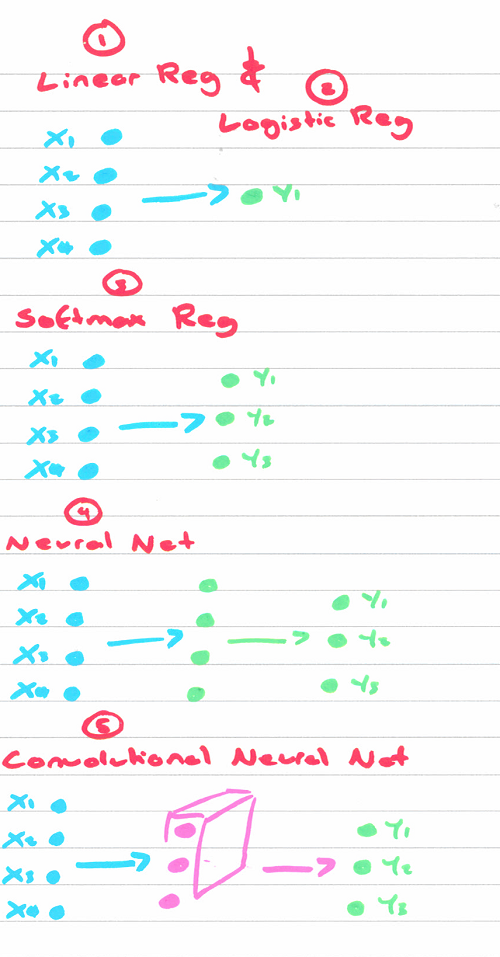### 一、线性回归（见笔记(github-ipynb)）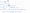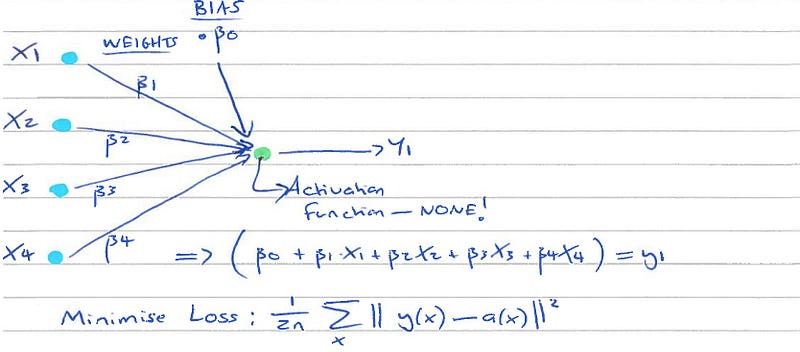# Matrix of explanatory variables
X <- as.matrix(X)
# Add column of 1s for intercept coefficient
intcpt <- rep(1, length(y))
# Combine predictors with intercept
X <- cbind(intcpt, X)
# OLS (closed-form solution)
beta_hat <- solve(t(X) %*% X) %*% t(X) %*% y

• 从随机地猜测权重开始
• 将所猜测的权重值代入损失函数中
• 将猜测值移向梯度的相反方向移动一小步（即我们所谓的“学习频率”）
• 重复上述步骤 N 次

GD 仅仅使用了 Jacobian 矩阵 (而不是 Hessian 矩阵)，不过我们知道， 当我们的损失函数为凸函数时，所有的极小值即（局部最小值）为（全局）最小值，因此 GD 总能够收敛至全局最小值。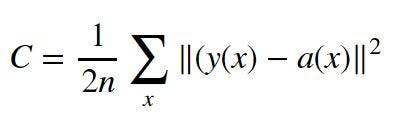# Start with a random guess
beta_hat <- matrix(0.1, nrow=ncol(X_mat))
# Repeat below for N-iterations
for (j in 1:N)
{
# Calculate the cost/error (y_guess - y_truth)
residual <- (X_mat %*% beta_hat) - y
# Calculate the gradient at that point
delta <- (t(X_mat) %*% residual) * (1/nrow(X_mat))
# Move guess in opposite direction of gradient
beta_hat <- beta_hat - (lr*delta)
}

200次的迭代之后我们会得到和闭包方法一样的梯度与参数。除了这代表着我们的进步意外（我们使用了 GD），这个迭代方法在当闭包方法因矩阵过大，而无法计算矩阵的逆的时候，也非常有用（因为有内存的限制）。

### 第二步 - 逻辑回归 (见笔记(github-ipynb))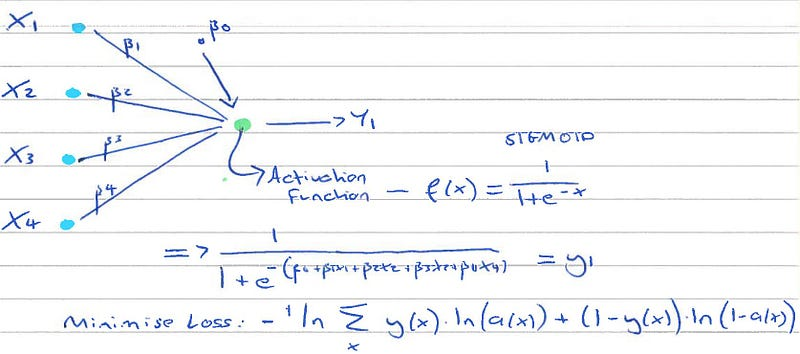1. 我们使用一种称为 logistic-sigmoid 的 ‘激活’/链接函数来将输出压缩至 0 到 1 的范围内
2. 不是最小化损失的方差而是最小化伯努利分布的负对数似然

sigmoid <- function(z){1.0/(1.0+exp(-z))}

log_likelihood <- function(X_mat, y, beta_hat)
{
scores <- X_mat %*% beta_hat
ll <- (y * scores) - log(1+exp(scores))
sum(ll)
}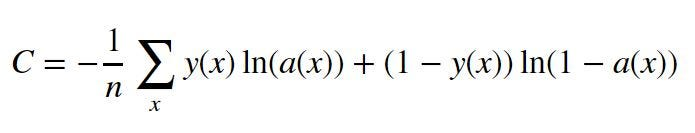logistic_reg <- function(X, y, epochs, lr)
{
X_mat <- cbind(1, X)
beta_hat <- matrix(1, nrow=ncol(X_mat))
for (j in 1:epochs)
{
# For a linear regression this was:
# 1*(X_mat %*% beta_hat) - y
residual <- sigmoid(X_mat %*% beta_hat) - y
# Update weights with gradient descent
delta <- t(X_mat) %*% as.matrix(residual, ncol=nrow(X_mat)) *  (1/nrow(X_mat))
beta_hat <- beta_hat - (lr*delta)
}
# Print log-likliehood
print(log_likelihood(X_mat, y, beta_hat))
# Return
beta_hat
}

### 三、Softmax 回归函数（无笔记）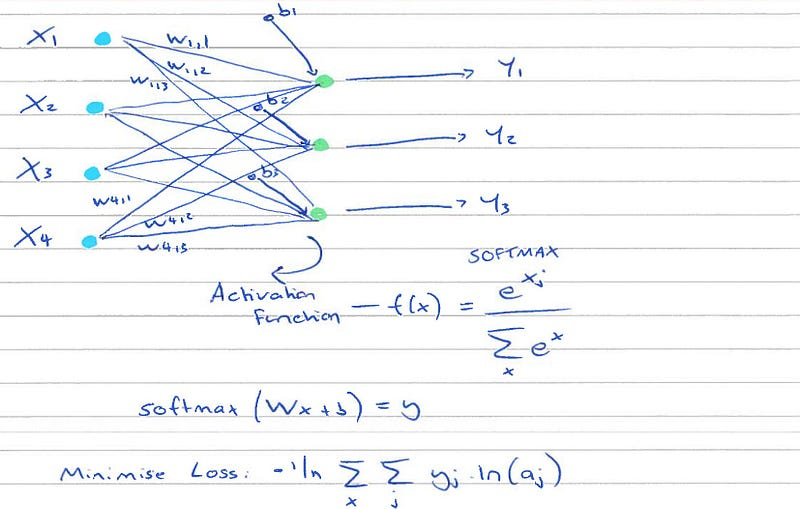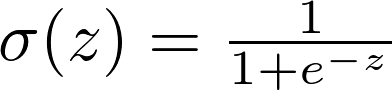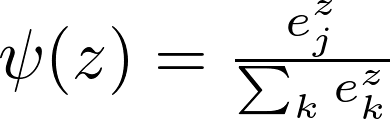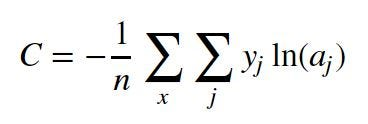（译者注：one-hot是将分类的特征转化为更加适合分类和回归算法的数据格式（Quora-Håkon Hapnes Strand），中文资料可见此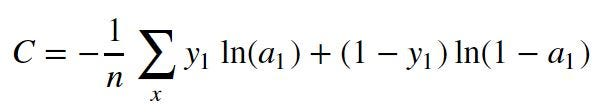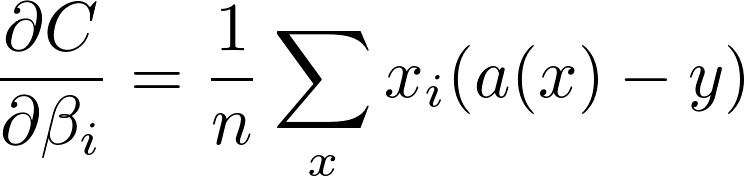### 四、神经网络（见笔记(github-ipynb))）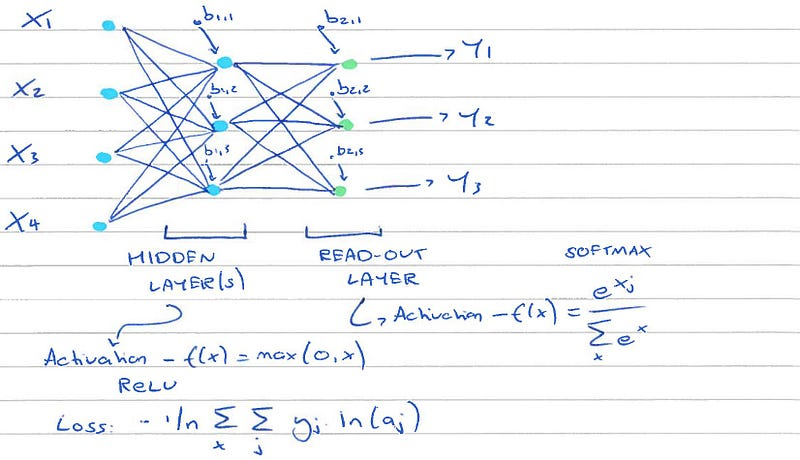1. 我们首先初始化权重：

neuralnetwork <- function(sizes, training_data, epochs, mini_batch_size, lr, C, verbose=FALSE, validation_data=training_data)

biases <- lapply(seq_along(listb), function(idx){
r <- listb[[idx]]
matrix(rnorm(n=r), nrow=r, ncol=1)
})

weights <- lapply(seq_along(listb), function(idx){
c <- listw[[idx]]
r <- listb[[idx]]
matrix(rnorm(n=r*c), nrow=r, ncol=c)
})
1. 我们使用随机梯度下降（SGD）作为我们的优化方法：
    SGD <- function(training_data, epochs, mini_batch_size, lr, C, sizes, num_layers, biases, weights,verbose=FALSE, validation_data)
{
# Every epoch
for (j in 1:epochs){
# Stochastic mini-batch (shuffle data)
training_data <- sample(training_data)
# Partition set into mini-batches
mini_batches <- split(training_data,
ceiling(seq_along(training_data)/mini_batch_size))
# Feed forward (and back) all mini-batches
for (k in 1:length(mini_batches)) {
# Update biases and weights
res <- update_mini_batch(mini_batches[[k]], lr, C, sizes, num_layers, biases, weights)
biases <- res[]
weights <- res[[-1]]
}
}
# Return trained biases and weights
list(biases, weights)
}
1. 作为 SGD 方法的一部分，我们更新了

 update_mini_batch <- function(mini_batch, lr, C, sizes, num_layers, biases, weights)
{
nmb <- length(mini_batch)
listw <- sizes[1:length(sizes)-1]
listb <-  sizes[-1]

# Initialise updates with zero vectors (for EACH mini-batch)
nabla_b <- lapply(seq_along(listb), function(idx){
r <- listb[[idx]]
matrix(0, nrow=r, ncol=1)
})
nabla_w <- lapply(seq_along(listb), function(idx){
c <- listw[[idx]]
r <- listb[[idx]]
matrix(0, nrow=r, ncol=c)
})

# Go through mini_batch
for (i in 1:nmb){
x <- mini_batch[[i]][]
y <- mini_batch[[i]][[-1]]
# Back propagation will return delta
# Backprop for each observation in mini-batch
delta_nablas <- backprop(x, y, C, sizes, num_layers, biases, weights)
delta_nabla_b <- delta_nablas[]
delta_nabla_w <- delta_nablas[[-1]]
# Add on deltas to nabla
nabla_b <- lapply(seq_along(biases),function(j)
unlist(nabla_b[[j]])+unlist(delta_nabla_b[[j]]))
nabla_w <- lapply(seq_along(weights),function(j)
unlist(nabla_w[[j]])+unlist(delta_nabla_w[[j]]))
}
# After mini-batch has finished update biases and weights:
# i.e. weights = weights - (learning-rate/numbr in batch)*nabla_weights
weights <- lapply(seq_along(weights), function(j)
unlist(weights[[j]])-(lr/nmb)*unlist(nabla_w[[j]]))
biases <- lapply(seq_along(biases), function(j)
unlist(biases[[j]])-(lr/nmb)*unlist(nabla_b[[j]]))
# Return
list(biases, weights)
}
2. 我们用来计算 delta 的算法是反向传播算法。

cost_delta <- function(method, z, a, y) {if (method=='ce'){return (a-y)}}

# Calculate activation function
sigmoid <- function(z){1.0/(1.0+exp(-z))}
# Partial derivative of activation function
sigmoid_prime <- function(z){sigmoid(z)*(1-sigmoid(z))}

backprop <- function(x, y, C, sizes, num_layers, biases, weights)

### 五、卷积神经网络（[见笔记(github.com/ilkarman/De…)]）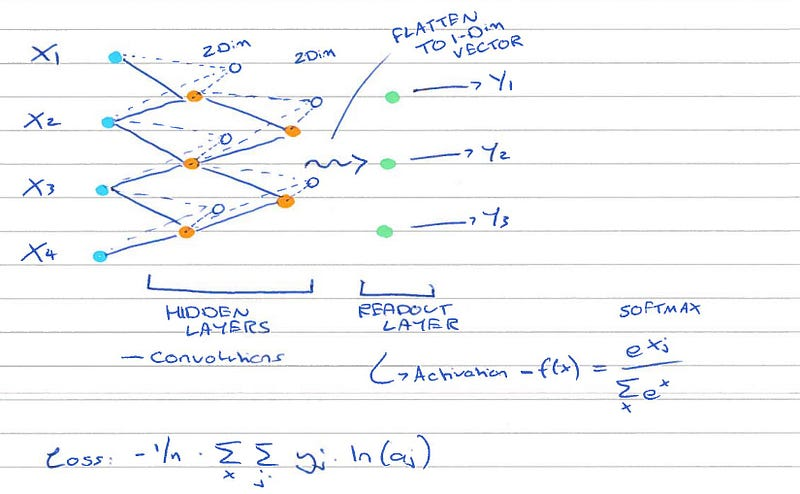CNN 卷积神经网络用一种很有趣的方式看待图像（提取特征）。开始时，他们只观察图像的很小一部分（每次），比如说一个大小为 5*5 像素的框（一个过滤器）。2D 用于图像的卷积，是将这个框扫遍整个图像。这个阶段会专门用于提取颜色和线段。然而，下一个神经层会转而关注之前过滤器的结合，因而‘放大来观察’。在一定数量的层数之后，神经网络会放的足够大而能识别出形状和更大的结构。

convolution <- function(input_img, filter, show=TRUE, out=FALSE)
{
conv_out <- outer(
1:(nrow(input_img)-kernel_size[]+1),
1:(ncol(input_img)-kernel_size[]+1),
Vectorize(function(r,c) sum(input_img[r:(r+kernel_size[]-1),
c:(c+kernel_size[]-1)]*filter))
)
}

conv_emboss <- matrix(c(2,0,0,0,-1,0,0,0,-1), nrow = 3)
convolution(input_img = r_img, filter = conv_emboss)

conv_sharpen <- matrix(c(0,-1,0,-1,5,-1,0,-1,0), nrow = 3)
convolution(input_img = r_img, filter = conv_sharpen)

filter_map <- lapply(X=c(1:64), FUN=function(x){
# Random matrix of 0, 1, -1
conv_rand <- matrix(sample.int(3, size=9, replace = TRUE), ncol=3)-2
convolution(input_img = r_img, filter = conv_rand, show=FALSE, out=TRUE)
})

square_stack_lst_of_matricies <- function(lst)
{
sqr_size <- sqrt(length(lst))
# Stack vertically
cols <- do.call(cbind, lst)
# Split to another dim
dim(cols) <- c(dim(filter_map[])[],
dim(filter_map[])[]*sqr_size,
sqr_size)
# Stack horizontally
do.call(rbind, lapply(1:dim(cols), function(i) cols[, , i]))
}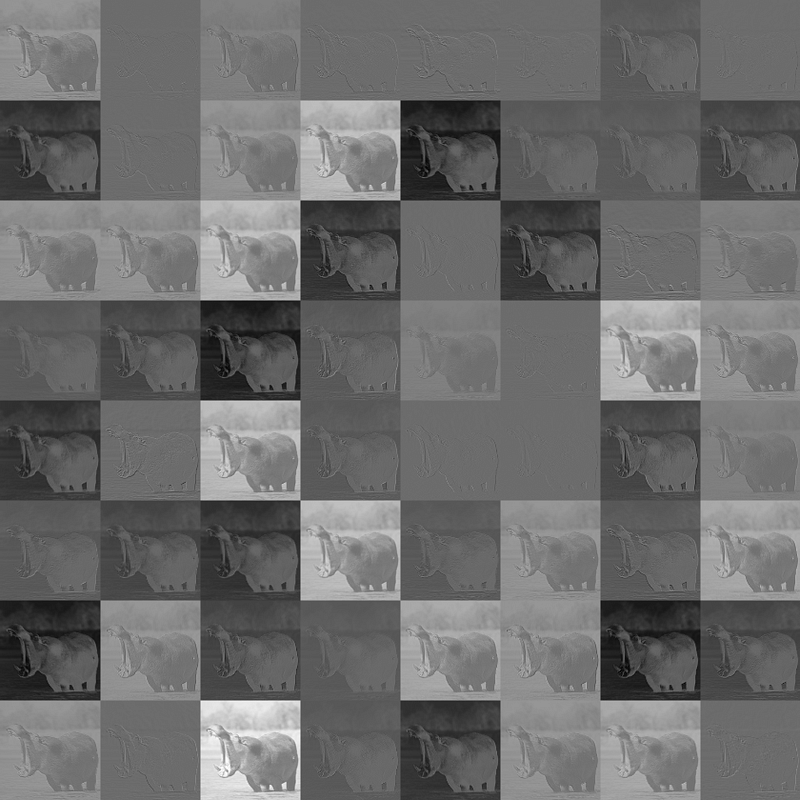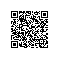使用钉钉扫一扫加入圈子
+ 订阅